Question
1 views

check_circle

Definition

The discrete-time convolution is for the discrete signals. it can be better understood as explained below.

Consider a unit impulse discrete  signal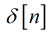. The impulse response of the the given discrete signal is given as: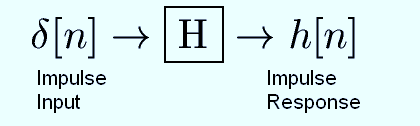The impulse signal is shown below: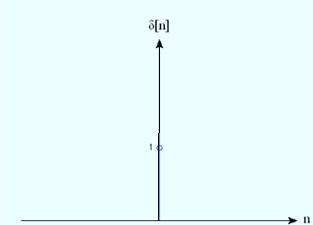The response of this signal is given below: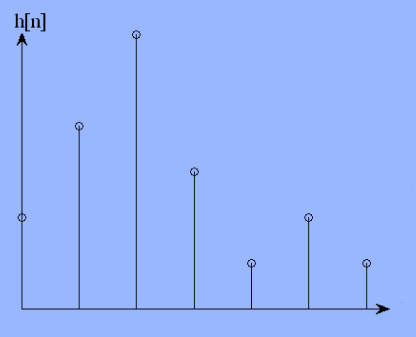description

if the input is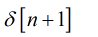the response will be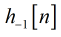. the response is shown below.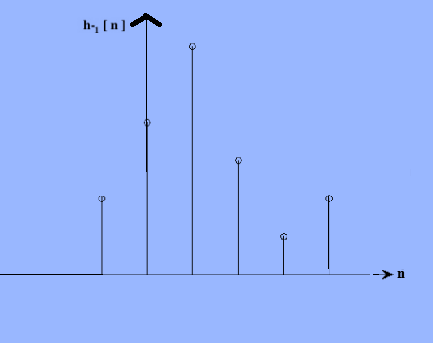from the above if the input is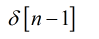the response will be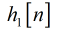.

the graph will be two units forward.

based on this the response can be related as: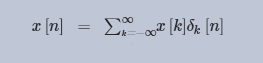...

### Want to see the full answer?

See Solution

#### Want to see this answer and more?

Solutions are written by subject experts who are available 24/7. Questions are typically answered within 1 hour.*

See Solution
*Response times may vary by subject and question.
Tagged in

### Electrical Engineering